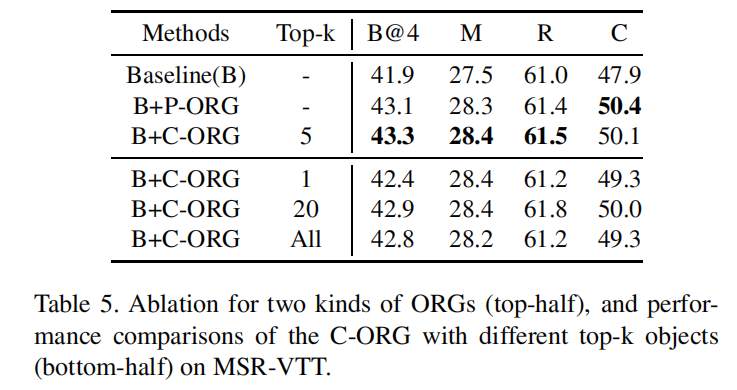Object Relational Graph with Teacher-Recommended Learning for Video Captioning论文笔记

[TOC]

整体框架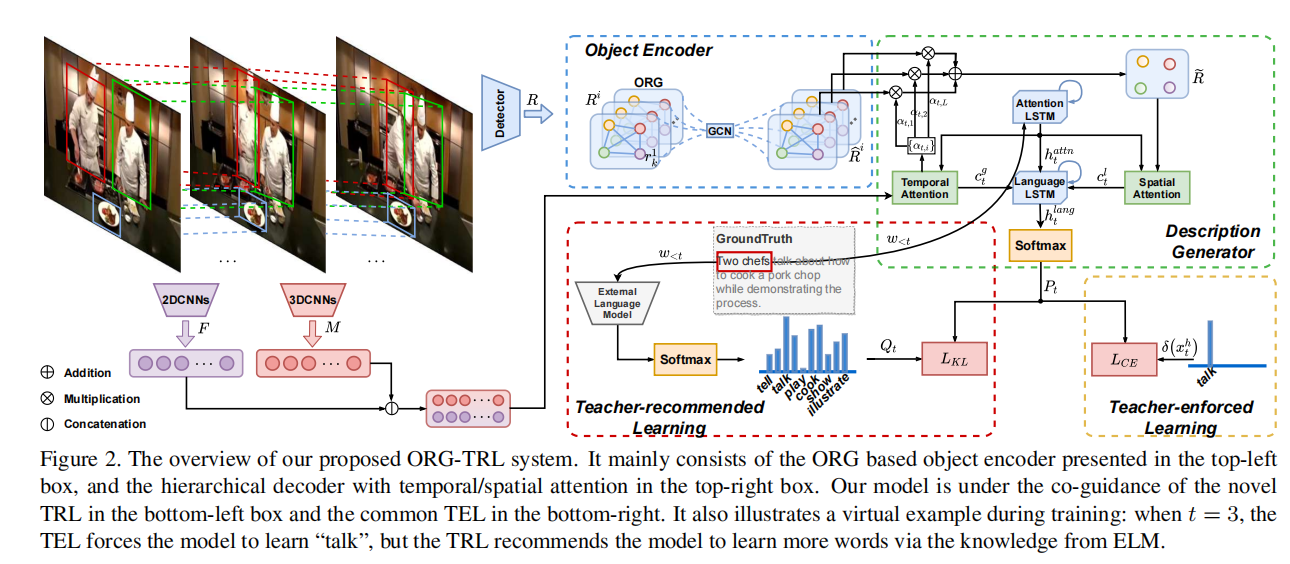Object Relational Graph编码器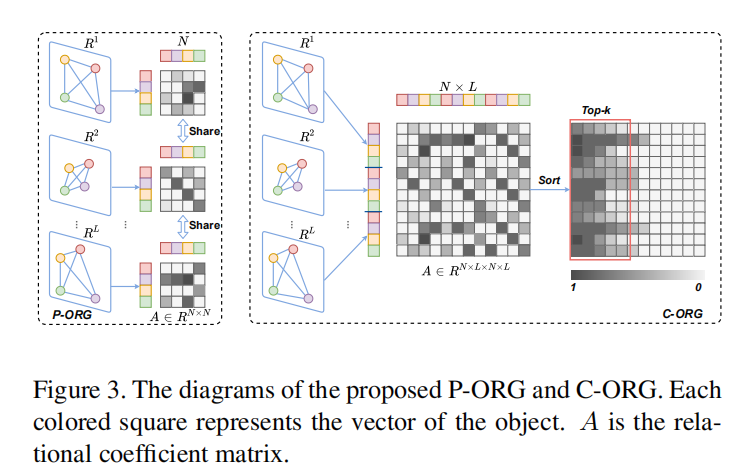Description Generation解码器

attn解码器使用LSTM网络，$\overline v$是降维掉时间轴的全局视频特征，$w_{t-1}$是前一个生成的单词，$h^{lang}_{t-1}$是language解码器的上一步hidden变量。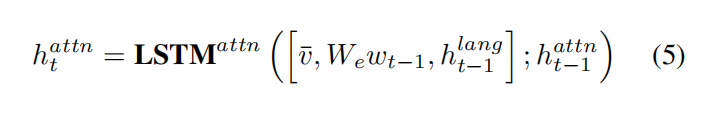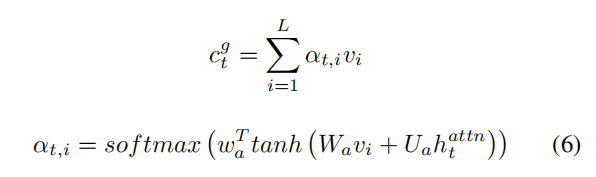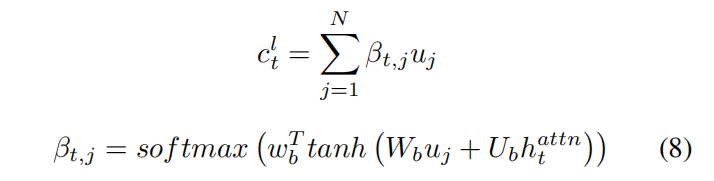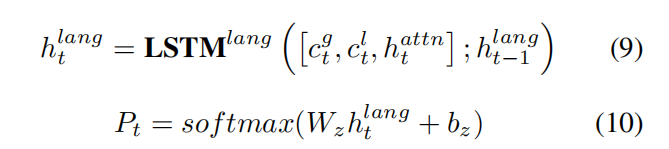Teacher Recommend Learning（TRL）

$$P_t=CAP(w_{<t}|\theta_{CAP}) \ \mathcal L_{CE} = -\sum^{T}_{t=1}\delta(x^h_t)^T \cdot logP_t$$

$$Q_t=ELM(w_{<t},T_e|\theta_{ELM})$$

$$\mathcal L_{KL} = -\sum^{T}{t=1} \sum{d} Q^d_t \cdot log P^d_t$$

实验结果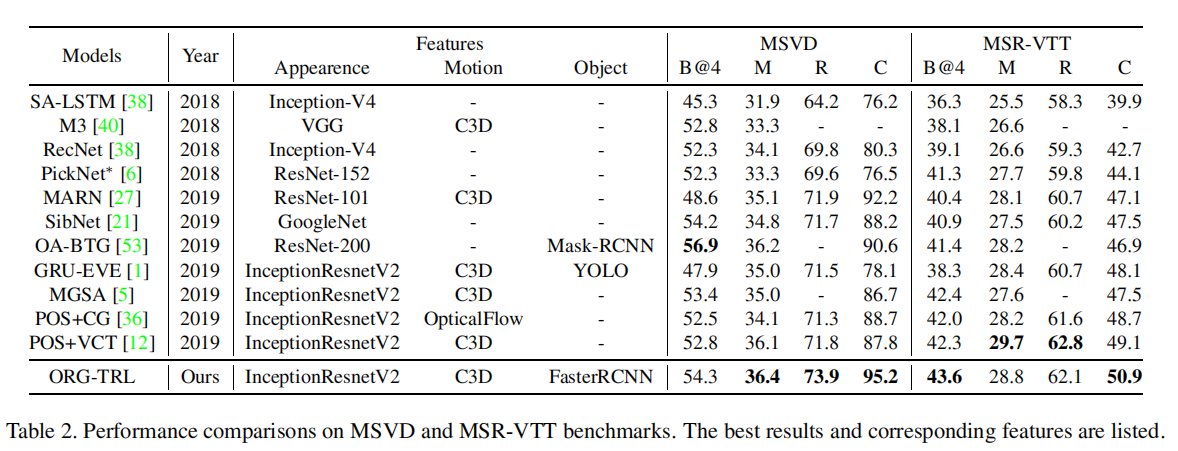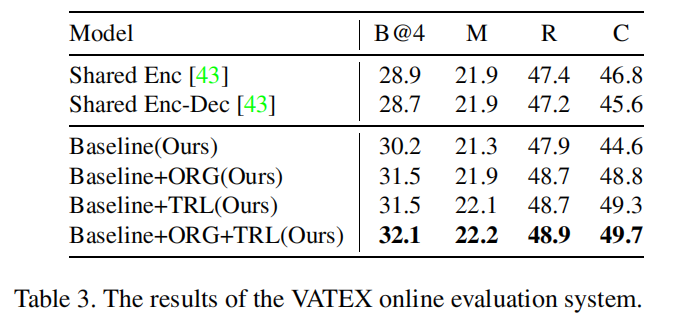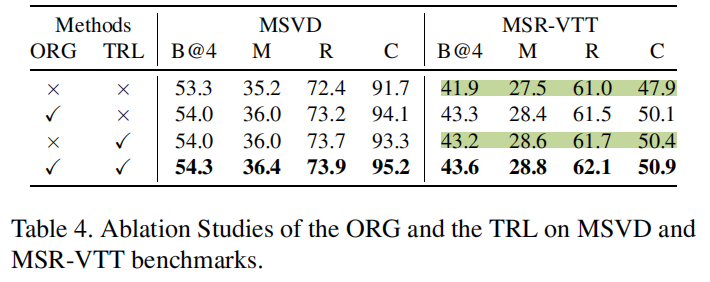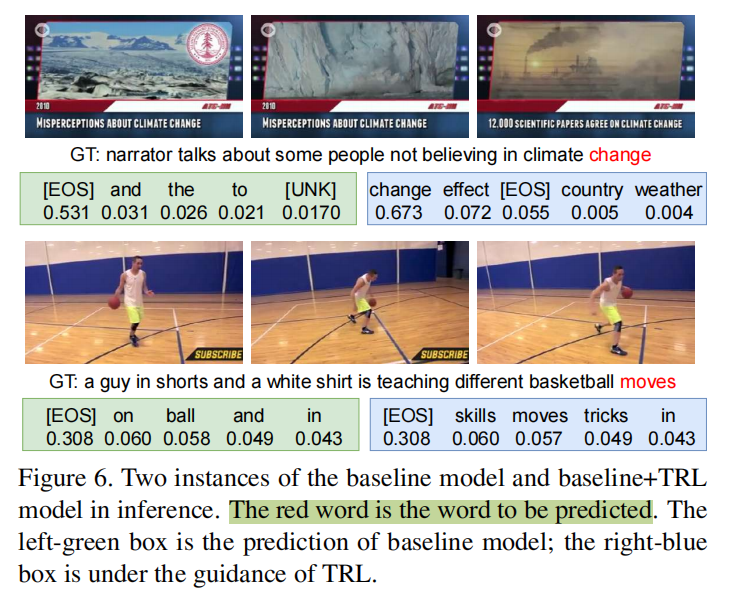Table3和Table4可以看出TRL贡献了非常多，超过了ORG部分的贡献量。Figure 6也很直观让人感受到TRL的厉害hh。TRL算是在最后的决策部分结合大规模预训练语言模型，效果显著。